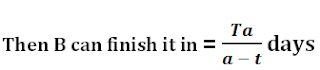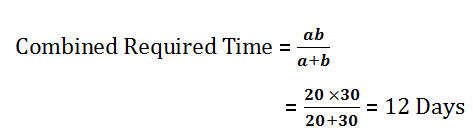# Time and Work - Concept with Practice Questions

Time and Work is an important chapter for banking exams. Technique used in this chapter can be implemented in various other chapters like Pipes and cisterns, Alligations etc.

### Case 1 - (If a person "A'' can do a job in 3 days)### Case 2 - (If a person "A'' does 2/7th work in one day)### Case 3 - (If a person "A" can do a job in 'a' days and "B" can do it in 'b' days)### Case 4 - (If a person "A" can do a job in 'a' days & A + B together can do it in T days)### Case 5 - (If A, B and C are working on a job then (A + B + C)'s one day work)

One day work of A + B + C = A's 1 Day Work + B's 1 Day Work + C's 1 Day Work

### Case 6 - (If "A" is twice as good worker as B)

In such a case following formula is applied:-Ques 1.   T and R can do a job alone in 20 days and 30 days respectively. In how many days the job will be finished if they work together?
a) 10 days
b) 11 days
c) 12 days
d) 15 days
e) None of The Days

Solution
(Option C)Ques 2. Maju and Sonu can do a job in 12 days. Sonu alone can finish it in 28 days. In how many days can Maju alone finish the work?
a) 19 days
b) 21 days
c) 20 days
d) 22 days
e) None of The Above

Solution
(Option B)
(Maju + Sonu)'s 1 day work  =  Maju's 1 day work + Sonu's 1 day work
1/12  =  Maju's 1 day work + 1/28
Maju's one day work = 1/12 - 1/28 =  1/21 th of work
Maju's alone time = {1 /  (1/21)} = 21 days

Ques 3. Mary and Maurice can do a piece of work in 10 days and 15 days respectively. They work together for 3 days and then Maurice leaves. Mary finishes the remaining work alone. In how many days is the total work finished?
a) 6 days
b) 5 days
c) 7 days
d) 8 days
e) None of The Above

Solution
(Option D)
Let the total work be finished in "T" days.
No. of days Mary worked / alone time + No. of days Maurice worked / alone time = 1
T  / 10 + 3 / 15 = 1
T / 10 = 1 - 1/ 15
T = 10 × 4 / 5 = 8
Total work is finished in 8 days

Ques 4. A, B and C can do a work in 10, 12 and 15 days respectively. If B leaves after 2 days, how long would it take A and C to complete the remaining work?
a) 3
b) 4
c) 5
d) 6
e) None of The Above

Solution
(Option A)
Let the total job is completed in T days.
No. of days of work / alone time = Part of work done
A's work + B's work + C's work = 1
T / 10 + 2 / 12 + T / 15 = 1
× 1 / 6 = 5 / 6
T = 5
Hence A and C takes 5 - 2 = 3 days to complete the rest job.

Ques 5.  Prince can do a work in 12 days. Queen works 60% more faster than Prince, then in how many days Queen alone would finish the work?
a) 14/2 days
b) 15/2 days
c) 17/2 days
d) 18/2 days
e) None of The Above

Solution
(Option B)
Prince's 1 day work  =  1 / 12
Queen is 60% more faster than Prince, So Queen's 1 day work = 1.6 ×  1 / 12 = 2 / 15
Queen can finish alone in {1 / (2 / 15)} = 15 /2 days

Ques 6. Sanskrit can do 3/4th part of a work in 15 days and Master completes the res part of work in 3 days, in how many days Sanskrit and Master together can finish the work?
a) 15/2 days
b) 13/2 days
c) 11/2 days
d) 9/2 days
e) None of The Above

Solution
(Option A)
Sanskrit's 1 day work = {(3/4) / 15} = 1/20
Master in 3 days completes rest 1/4th the work
Master's 1 day work = {(1/4) / 3} = 1/12
(Sanskrit + Master)'s 1 day work = 1 /20 + 1 / 12  =  8 / 60
Time for completion = 1 / 1 day's combined work
Required time for ( Sanskrit + Master) =  60  /  8  =  15 / 2 days

Ques 7. Ramesh is thrice as good workman as Bipan, and is therefore able to finish a piece of work in 40 days less than Bipan, find the time for both together to complete the work?
a) 17 days
b) 18 days
c) 19 days
d) 15 days
e) None of The Above

Solution
(Option D)
Since Ramesh is thrice as good workman as Bipan. So, if Bipan completes in 3t days, then Ramesh does in t days
As per question,
3t - t = 40
t = 20
Bipan takes 60 days and Ramesh takes 20 days (Ramesh + Bipan)'s 1 day work
1 / 60 + 1 / 20 = 1 / 15
Time for completion =  1 / 1 day's combined work = 1 / (1/15) = 15 days

Ques 8. Akshay and Sunig working separately can assemble a computer in 10 hours and 12 hours respectively. If they are working for 1 hour alternately, Akshay beginning, in how many hours will the computer be assembled?
a) 65 / 6 hours
b) 55 / 6 hours
c) 45 / 6 hours
d) 35 / 6 hours
e) None of The Above

Solution
(Option A)
After 10 hours of combined, but alternate working, we get the part of computer assembled = Akshay's 5 hours work + Sunil's 5 hours work
= 5 × 1 /10 + 5 × 1 / 12
= 11 / 12 th
Remaining part = 1 - 11 / 12 = 1 / 12th
Now, at the start of 11th hour, Akshay will work
Time taken by Akshay to do 1 / 12th work = 10 × 1 / 12 hour = 5 / 6 hour
Total time = 10 hours + 5 / 6 hours = 10(5/6) hours
the computer will be assembled in 65 / 6 hours.

Ques 9. Two friends take a piece of work for Rs. 960. One alone could do it in 12 days, the other in 16 days. With the assistance of an expert they finish it in 4 days. How much remuneration the expert should get?
a) Rs. 400
b) Rs. 500
c) Rs. 800
d) Rs. 1000
e) None of The Above

Solution
(Option A)
First Friend's 4 day's work = 4 / 12 = 1 / 3 (Since, the work is finished in 4 days, when expert assists)
Second Friend's 4 day's work = 4 / 16 = 1 / 4
The expert's 4 day's work = 1 - (1/3 + 1/4) = 5 / 12
Now, total wages of Rs. 900 is to be distributed among two friends and the expert in proportion to the amount of work done by each of them.
So, 960 is to be divided in the proportion of   1/3 :  1/4  :  5 /12  or  4 : 3 : 5
Share of Expert = 5 / 12 ×  960 = Rs. 400
Hence, the expert should get Rs. 400.

Ques 10. One Thousand men in a fortress have provisions for 12 days. How long will the provisions last if 200 more men join them?
a) 8 days
b) 10 days
c) 12 days
d) 15 days
e) None of The Above

Solution
(Option B)
Here amount of work, i.e. amount of provisions is same
1000 × 12 = (1000 + 200) × D2
D2 = 10 days